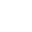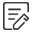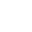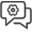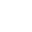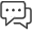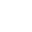RECOMMEND NEWS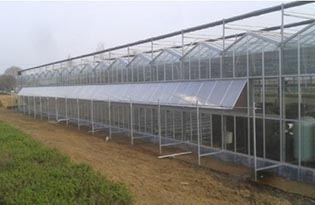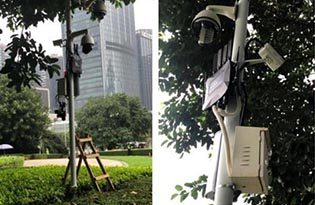FEATURED PRODUCT

Date:2022-06-07 17:10:39

### What does DBI mean? Detailed Introduction of DBI in Wireless Routers

DBI and dBd are units of power gain, both are relative values, but the reference is not the same. The reference reference for dBi is an omnidirectional antenna; the reference reference for dBd is a dipole. It is generally believed that dBi and dBd represent the same gain, and the value expressed in dBi is 2.15 larger than the value expressed in dBd. (ie dBi=dBd+2.15). G(dBi)=10lgGi G(dBd)=10lgGd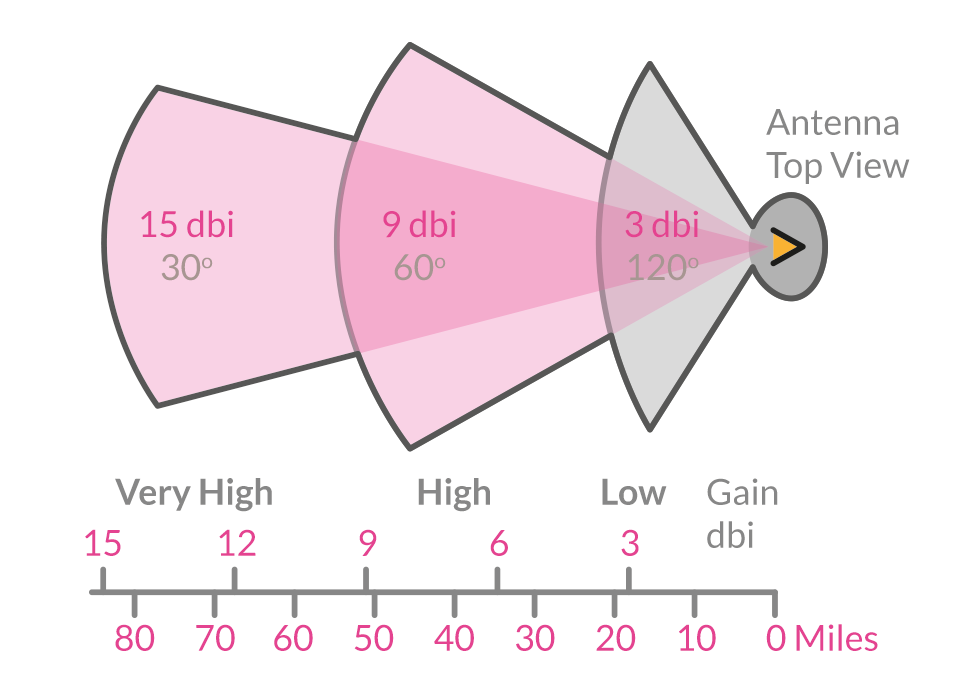1. What does dBi mean

1.1 For an antenna with a gain of 16dBd, when its gain is converted into dBi, it is 18.15dBi (generally ignoring the decimal place, it is 18dBi).

1.2 GSM900 antenna gain can be 13dBd (15dBi), GSM1800 antenna gain can be 15dBd (17dBi).
dB is also a unit of power gain and represents a relative value. When calculating how many dB the power of A is larger or smaller than that of B, it can be calculated according to the formula 10 lg A/B. For example: A power is twice as large as B power, then 10 lg A/B = 10 lg 2 = 3dB. That is to say, the power of A is 3dB greater than that of B; if the power of A is 46dBm and the power of B is 40dBm, then it can be said that A is 6dB greater than that of B; if the antenna A is 12dBd and the antenna B is 14dBd, it can be said that A is 2dB smaller than B.
dBm is a value that examines the absolute value of power. The calculation formula is: power and P (watt) conversion formula: P'dBm=10lg(P/1mW) (P: watt; P': unit is dbm).

1.3 If the transmit power P is 1mw, it is 0dBm after converting to dBm.

1.4 For 40W power, the converted value in dBm should be:
10lg(40W/1mW) = 10lg(40000) = 10(4+lg4) = 46dBm.

Sometimes you will also see dBc, which is also a unit that expresses the relative value of power, and is calculated in exactly the same way as dB. Generally speaking, dBc is relative to the carrier power. In many cases, it is used to measure the relative value of the carrier power, such as measuring interference (co-channel interference, intermodulation interference, intermodulation interference, band external interference, etc.) and the relative magnitude of coupling, spurious, etc. Where dBc is used, dB can in principle also be used instead.

In the parameters of wireless routers, it is often seen that the unit of antenna gain is dBi, and the common parameters are 3dBi, 5dBi or 7dBi. And many netizens don't know what dBi means? Today, the editor will talk about what does dBi mean for wireless routers? Tell me the meaning of this value and the more often asked dBi value, the bigger the better or the smaller the better?

2. What does dBi mean in wireless routers

In wireless routers, the antenna gain is also an important parameter, which is related to the router's transmission distance and wall penetration capability, and dBi is the gain unit of the wireless antenna. To put it simply, in a wireless router, dBi is a unit used to mark the antenna gain of a router, which is one of the professional terms in the term router.

At present, most of the common wireless routers are 3dBi, 5dBi or 7dBi by looking at the parameters. For users, is this value as large as possible, or as small as possible? For this problem, in fact, through the following antenna gain diagram to see Understand in seconds.

In the case of the same wireless transmission power of the router, the larger the dBi value, the higher the gain, the smaller the vertical angle, and the longer the transmission distance; but the short-range transmission area is small, and the smaller the dBi value, the smaller the gain, the smaller the vertical angle. The higher the transmission distance, the larger the radiation area at close range.

At present, the common wireless router antenna gain values ​​on the market are generally 3dBi and 5dBi, while some main through-wall router antenna gains use 7dBi gain wireless antennas. For ordinary users, if the home area is not very large, the gain of 3-5dBi is beneficial to signal reception. If you want to increase the antenna, although the ability to penetrate the wall is enhanced, the covered area is often more prone to signal dead ends. Such routers often need to use more antennas to solve the signal coverage problem, and the product price is often a lot more expensive.

Previous Page:What is 5G WiFi Range? 5Ghz WiFi Router Range Standard

next page:How to Install a New 4G LTE WiFi Modem